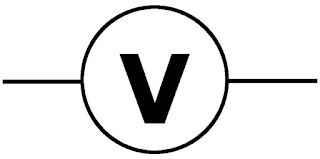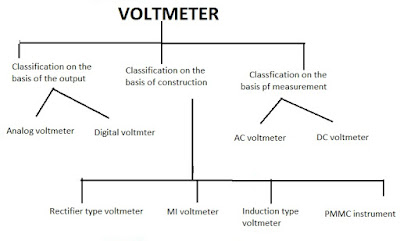## Definition of voltmeter?

A voltmeter is also known as the voltage meter. It is an instrument that measures the voltage or potential difference in voltage known as the voltmeter. As we know, The voltmeter is always connected in parallel with the circuit. Some voltmeter is intended for the direct current called as a DC circuit and other designed for alternating current called the AC circuit.

It works on the basic principle that the torque deflects the pointer of the instrument. Thus defection of the pointer is directly proportional to the potential difference between the points.

## Symbolic represantation of voltmeter?Voltmeter symbol

## Type of voltmeter:Voltmeter types

1. Classification  on the basis of Output:

• Analog voltmeter
The analog voltmeter uses for measuring the AC voltage. An analog voltmeter consists of a sensitive galvanometer in the series with high resistance. In this type, the internal resistance of a voltmeter must be high. The sensitivity of the galvanometer and the value of the series resistance determine the range of voltage that the meter can display.

• Digital voltmeter
The voltmeter which displays the values or reading in the numeric form is known as the digital voltmeter. The digital voltmeter gives an accurate result.

2. Classification on the basis of construction

• Rectifier type voltmeter
This type of instrument is used as the AC circuit for voltage measurement. The rectifier instrument converts the AC quantity into the DC quantity with the help of the rectifier. And this can the DC signal is measured the PMMC instrument.
• MI voltmeter
MI instrument means that moving iron instrument. It is used for both AC and DC measurements, just because the deflection proportional to the square of the voltage assuming impedance of the meter to constant, so whatever is the polarity of the voltage it shows directional defection.

The moving iron instrument is classified into two categories:
• Attraction type moving iron instrument
• Repulsion type moving iron instrument

• PMMC voltmeter

This voltmeter can work on the principle that the current-carrying conductor placed in the magnetic field and because of the current force acting on the conductor. The current induces in the PMMC because of the measured voltage, and this current deflects the pointer of the meter.

3. Classification on the basis of Measurement

The voltmeter which measures the direct current is known as the DC voltmeter and is used in the AC circuit for alternating voltage measurement called  AC voltmeter.

## Definition of voltmeter?

A voltmeter is also known as the voltage meter. It is an instrument that measures the voltage or potential difference in voltage known as the voltmeter. As we know, The voltmeter is always connected in parallel with the circuit. Some voltmeter is intended for the direct current called as a DC circuit and other designed for alternating current called the AC circuit.

It works on the basic principle that the torque deflects the pointer of the instrument. Thus defection of the pointer is directly proportional to the potential difference between the points.

## Symbolic represantation of voltmeter?Voltmeter symbol

## Type of voltmeter:Voltmeter types

1. Classification  on the basis of Output:

• Analog voltmeter
The analog voltmeter uses for measuring the AC voltage. An analog voltmeter consists of a sensitive galvanometer in the series with high resistance. In this type, the internal resistance of a voltmeter must be high. The sensitivity of the galvanometer and the value of the series resistance determine the range of voltage that the meter can display.

• Digital voltmeter
The voltmeter which displays the values or reading in the numeric form is known as the digital voltmeter. The digital voltmeter gives an accurate result.

2. Classification on the basis of construction

• Rectifier type voltmeter
This type of instrument is used as the AC circuit for voltage measurement. The rectifier instrument converts the AC quantity into the DC quantity with the help of the rectifier. And this can the DC signal is measured the PMMC instrument.
• MI voltmeter
MI instrument means that moving iron instrument. It is used for both AC and DC measurements, just because the deflection proportional to the square of the voltage assuming impedance of the meter to constant, so whatever is the polarity of the voltage it shows directional defection.

The moving iron instrument is classified into two categories:
• Attraction type moving iron instrument
• Repulsion type moving iron instrument

• PMMC voltmeter

This voltmeter can work on the principle that the current-carrying conductor placed in the magnetic field and because of the current force acting on the conductor. The current induces in the PMMC because of the measured voltage, and this current deflects the pointer of the meter.

3. Classification on the basis of Measurement

The voltmeter which measures the direct current is known as the DC voltmeter and is used in the AC circuit for alternating voltage measurement called  AC voltmeter.